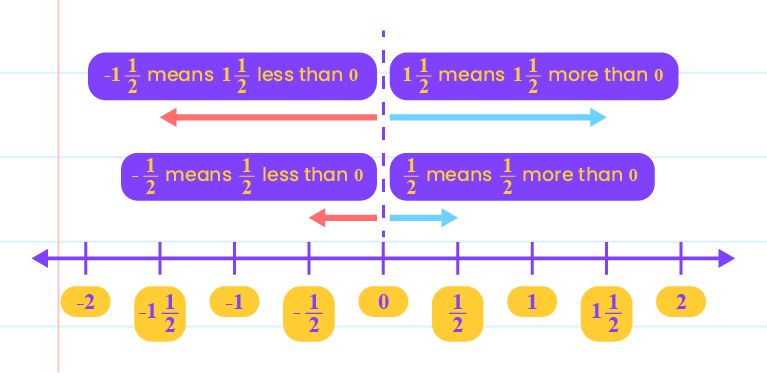## Positive and Negative Fractions

1.3  Positive and Negative Fractions

• Positive fractions are fractions more than $$0$$.
• Negative fractions are fractions less than $$0$$.• The values of two or more fractions can be compared by equating the denominator first.
• Then, arranged the fractions in ascending or descending order.

Combined basic arithmetic operations of positive and negative fractions:

 Example Solve: \begin{aligned} &\space1\dfrac{1}{6}\times\bigg(\dfrac{3}{4}-\dfrac{1}{5}\bigg) \\\\&=\dfrac{7}{6}\times\bigg(\dfrac{15-4}{20}\bigg) \\\\&=\dfrac{7}{6}\times\dfrac{11}{20} \\\\&=\dfrac{77}{120}. \end{aligned}